# Convective-Stratiform classification#

This example shows how to use the updated convective stratiform classifcation algorithm. We show 3 examples, a summer convective example, an example from Hurricane Ian, and an example from a winter storm.

```print(__doc__)

# Author: Laura Tomkins (lmtomkin@ncsu.edu)

import cartopy.crs as ccrs
import matplotlib.colors as mcolors
import matplotlib.pyplot as plt
import numpy as np

import pyart
```

## How the algorithm works#

This first section describes how the convective-stratiform algorithm works (see references for full details). This algorithm is a feature detection algorithm and classifies fields as “convective” or “stratiform”. The algorithm is designed to detect features in a reflectivity field but can also detect features in fields such as rain rate or snow rate. In this section we describe the steps of the convective stratiform algorithm and the variables used in the function. The first step of the algorithm calculates a background average of the field with a circular footprint using a radius provided by `bkg_rad_km`. A larger radius will yield a smoother field. The radius needs to be at least double the grid spacing, but we recommend at least three times the grid spacing. If using reflectivity, `dB_averaging` should be set to True to convert reflectivity to linear Z before averaging, False for rescaled fields such as rain or snow rate. `calc_thres` determines the minimum fraction of a circle that is considered in the background average calculation (default is 0.75, so the points along the edges where there is less than 75% of a full circle of data, the algorithm is not run). Once the background average has been calculated, the original field is compared to the background average. In order for points to be considered “convective cores” they must exceed the background value by a certain value or simply be greater than the `always_core_thres`. This value is determined by either a cosine scheme, or a scalar value (i.e. the reflectivity value must be X times the background value (multiplier; `use_addition=False`), or X greater than the background value (`use_addition=True`) where X is the `scalar_diff`). `use_cosine` determines if a cosine scheme or scalar scheme is to be used. If `use_cosine` is True, then the `max_diff` and `zero_diff_cos_val` come into use. These values define the cosine scheme that is used to determine the minimum difference between the background and reflectivity value in order for a core to be identified. `max_diff` is the maximum difference between the field and the background for a core to be identified, or where the cosine function crosses the y-axis. The `zero_diff_cos_val` is where the difference between the field and the background is zero, or where the cosine function crosses the x-axis. Note, if `always_core_thres` < `zero_diff_cos_val`, `zero_diff_cos_val` only helps define the shape of the cosine curve and all values greater than `always_core_thres` will be considered a convective core. If `always_core_thres` > `zero_diff_cos_val` then all values greater than `zero_diff_cos_val` will be considered a convective core. We plot some examples of the schemes below:

```# plot cosine scheme
pyart.graph.plot_convstrat_scheme(
always_core_thres=30, use_cosine=True, max_diff=5, zero_diff_cos_val=45
)
# when zero_diff_cos_val is greater than always_core_thres, the difference becomes zero at the zero_diff_cos_val
pyart.graph.plot_convstrat_scheme(
always_core_thres=55, use_cosine=True, max_diff=5, zero_diff_cos_val=45
)
# alternatively, we can use a simpler scalar difference instead of a cosine scheme
pyart.graph.plot_convstrat_scheme(
always_core_thres=40,
use_cosine=False,
max_diff=None,
zero_diff_cos_val=None,
scalar_diff=2,
)
# if you are interested in picking up weak features, you can also use the scalar difference as a multiplier instead,
# so very weak features do not have to be that different from the background to be classified as convective.
pyart.graph.plot_convstrat_scheme(
always_core_thres=40,
use_cosine=False,
max_diff=None,
zero_diff_cos_val=None,
scalar_diff=2,
)
```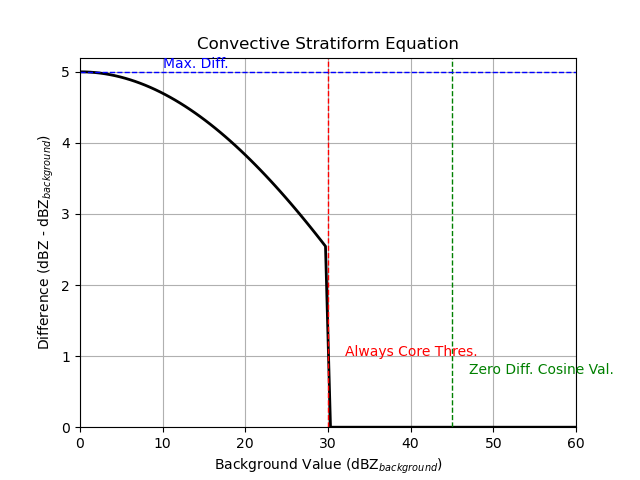Once the cores are identified, there is an option to remove speckles (`remove_small_objects`) smaller than a given size (`min_km2_size`). After the convective cores are identified, We then incorporate convective radii using `val_for_max_conv_rad` and `max_conv_rad_km`. The convective radii act as a dilation and are used to classify additional points around the cores as convective that may not have been identified previously. The `val_for_max_conv_rad` is the value where the maximum convective radius is applied and the `max_conv_rad_km` is the maximum convective radius. Values less than the `val_for_max_conv_rad` are assigned a convective radius using a step function. Finally, the points are classified as NOSFCECHO (threshold set with `min_dBZ_used`; 0), WEAKECHO (threshold set with `weak_echo_thres`; 3), SF (stratiform; 1), CONV (convective; 2).

## Examples#

Classification of summer convective example

Our first example classifies echo from a summer convective event. We use a cosine scheme to classify the convective points.

```# Now let's do a classification with our parameters
filename = pyart.testing.get_test_data("swx_20120520_0641.nc")

# extract the lowest sweep

# interpolate to grid
grid_shape=(1, 201, 201),
grid_limits=((0, 10000), (-50000.0, 50000.0), (-50000.0, 50000.0)),
fields=["reflectivity_horizontal"],
)

# get dx dy
dx = grid.x["data"] - grid.x["data"]
dy = grid.y["data"] - grid.y["data"]

# convective stratiform classification
convsf_dict = pyart.retrieve.conv_strat_yuter(
grid,
dx,
dy,
refl_field="reflectivity_horizontal",
always_core_thres=40,
use_cosine=True,
max_diff=5,
zero_diff_cos_val=55,
weak_echo_thres=10,
)

# mask zero values (no surface echo)
# mask 3 values (weak echo)

# create plot using GridMapDisplay
# plot variables
display = pyart.graph.GridMapDisplay(grid)
magma_r_cmap = plt.get_cmap("magma_r")
ref_cmap = mcolors.LinearSegmentedColormap.from_list(
"ref_cmap", magma_r_cmap(np.linspace(0, 0.9, magma_r_cmap.N))
)
projection = ccrs.AlbersEqualArea(
)
```

Note how the convective stratiform field has less data around the edges compared to the reflectivity field. This is because the function is designed to only compute the background radius where the footprint has 75% of data, so along the edges there is not enough data to fulfill this requirement. The footprint percentage can be changed using the variable `calc_thres`.

```# plot
plt.figure(figsize=(10, 4))
ax1 = plt.subplot(1, 2, 1, projection=projection)
display.plot_grid(
"reflectivity_horizontal",
vmin=5,
vmax=45,
cmap=ref_cmap,
transform=ccrs.PlateCarree(),
ax=ax1,
)
ax2 = plt.subplot(1, 2, 2, projection=projection)
display.plot_grid(
"convsf",
vmin=0,
vmax=2,
cmap=plt.get_cmap("viridis", 3),
ax=ax2,
transform=ccrs.PlateCarree(),
ticks=[1 / 3, 1, 5 / 3],
ticklabs=["", "Stratiform", "Convective"],
)
plt.show()
```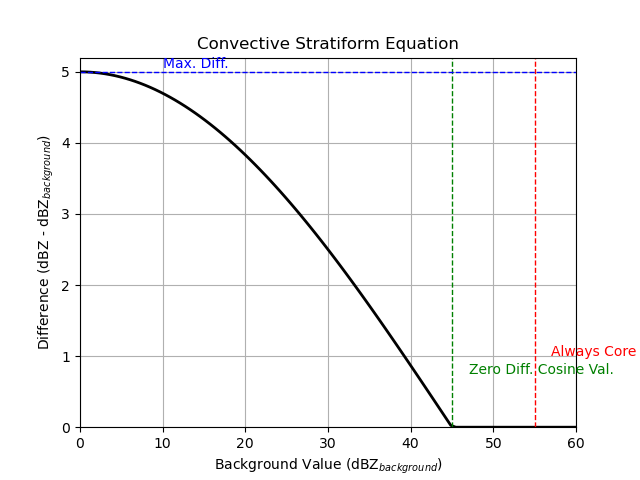In addition to the default convective-stratiform classification, the function also returns an underestimate (convsf_under) and an overestimate (convsf_over) to take into consideration the uncertainty when choosing classification parameters. The under and overestimate use the same parameters, but vary the input field by a certain value (default is 5 dBZ, can be changed with `estimate_offset`). The estimation can be turned off ( `estimate_flag=False`), but we recommend keeping it turned on.

```# mask weak echo and no surface echo
# underest.
# overest.

# Plot each estimation
plt.figure(figsize=(10, 4))
ax1 = plt.subplot(131)
ax1.pcolormesh(
convsf_dict["convsf"]["data"][0, :, :],
vmin=0,
vmax=2,
cmap=plt.get_cmap("viridis", 3),
)
ax1.set_title("Best estimate")
ax1.set_aspect("equal")
ax2 = plt.subplot(132)
ax2.pcolormesh(
convsf_dict["convsf_under"]["data"], vmin=0, vmax=2, cmap=plt.get_cmap("viridis", 3)
)
ax2.set_title("Underestimate")
ax2.set_aspect("equal")
ax3 = plt.subplot(133)
ax3.pcolormesh(
convsf_dict["convsf_over"]["data"], vmin=0, vmax=2, cmap=plt.get_cmap("viridis", 3)
)
ax3.set_title("Overestimate")
ax3.set_aspect("equal")
plt.show()
```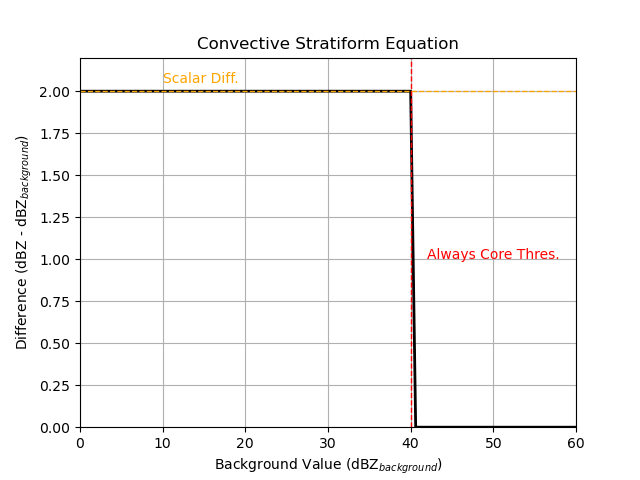Tropical example

Let’s get a NEXRAD file from Hurricane Ian

```# Read in file

# extract the lowest sweep

# interpolate to grid
grid_shape=(1, 201, 201),
grid_limits=((0, 10000), (-200000.0, 200000.0), (-200000.0, 200000.0)),
fields=["reflectivity"],
)

# get dx dy
dx = grid.x["data"] - grid.x["data"]
dy = grid.y["data"] - grid.y["data"]

# convective stratiform classification
convsf_dict = pyart.retrieve.conv_strat_yuter(
grid,
dx,
dy,
refl_field="reflectivity",
always_core_thres=40,
use_cosine=True,
max_diff=3,
zero_diff_cos_val=55,
weak_echo_thres=5,
estimate_flag=False,
)

# mask zero values (no surface echo)
# mask 3 values (weak echo)

# create plot using GridMapDisplay
# plot variables
display = pyart.graph.GridMapDisplay(grid)
magma_r_cmap = plt.get_cmap("magma_r")
ref_cmap = mcolors.LinearSegmentedColormap.from_list(
"ref_cmap", magma_r_cmap(np.linspace(0, 0.9, magma_r_cmap.N))
)
projection = ccrs.AlbersEqualArea(
)
# plot
plt.figure(figsize=(10, 4))
ax1 = plt.subplot(1, 2, 1, projection=projection)
display.plot_grid(
"reflectivity",
vmin=5,
vmax=45,
cmap=ref_cmap,
transform=ccrs.PlateCarree(),
ax=ax1,
axislabels_flag=False,
)
ax2 = plt.subplot(1, 2, 2, projection=projection)
display.plot_grid(
"convsf",
vmin=0,
vmax=2,
cmap=plt.get_cmap("viridis", 3),
axislabels_flag=False,
transform=ccrs.PlateCarree(),
ticks=[1 / 3, 1, 5 / 3],
ticklabs=["", "Stratiform", "Convective"],
ax=ax2,
)
plt.show()
```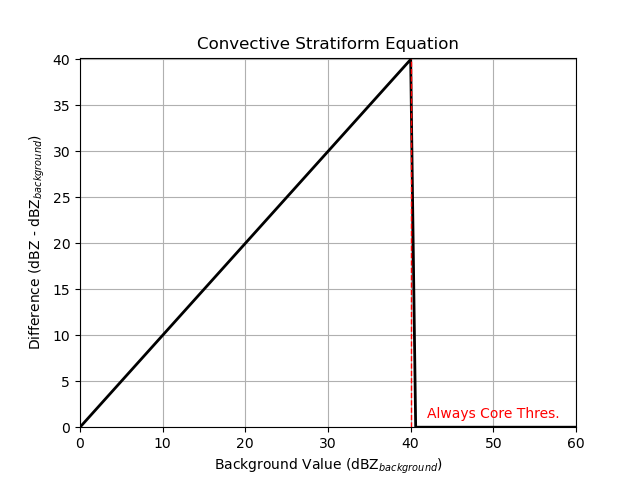Winter storm example with image muting

Here is a final example of the convective stratiform classification using an example from a winter storm. Before doing the classification, we image mute the reflectivity to remove regions with melting or mixed precipitation. We then rescale the reflectivity to snow rate (Rasumussen et al. 2003). We recommend using a rescaled reflectivity to do the classification, but if you do make sure to changed dB_averaging to False because this parameter is used to convert reflectivity to a linear value before averaging (set dB_averaging to True for reflectivity fields in dBZ units). In this example, note how we change some of the other parameters since we are classifying snow rate instead of reflecitivity.

```# Read in file

# extract the lowest sweep

# interpolate to grid
grid_shape=(1, 201, 201),
grid_limits=((0, 10000), (-200000.0, 200000.0), (-200000.0, 200000.0)),
fields=["reflectivity", "cross_correlation_ratio"],
)

# image mute grid object
grid, "reflectivity", "cross_correlation_ratio", 0.97, 20
)

# convect non-muted reflectivity to snow rate
nonmuted_ref = grid.fields["nonmuted_reflectivity"]["data"][0, :, :]

nonmuted_ref_linear = 10 ** (nonmuted_ref / 10)  # mm6/m3
snow_rate = (nonmuted_ref_linear / 57.3) ** (1 / 1.67)  #

snow_rate_dict = {
"data": snow_rate[None, :, :],
"standard_name": "snow_rate",
"long_name": "Snow rate converted from linear reflectivity",
"units": "mm/hr",
"valid_min": 0,
"valid_max": 40500,
}

# get dx dy
dx = grid.x["data"] - grid.x["data"]
dy = grid.y["data"] - grid.y["data"]

# convective stratiform classification
convsf_dict = pyart.retrieve.conv_strat_yuter(
grid,
dx,
dy,
refl_field="snow_rate",
dB_averaging=False,
always_core_thres=4,
use_cosine=True,
max_diff=1.5,
zero_diff_cos_val=5,
weak_echo_thres=0,
min_dBZ_used=0,
estimate_flag=False,
)

# mask zero values (no surface echo)
# mask 3 values (weak echo)

# create plot using GridMapDisplay
# plot variables
display = pyart.graph.GridMapDisplay(grid)
magma_r_cmap = plt.get_cmap("magma_r")
ref_cmap = mcolors.LinearSegmentedColormap.from_list(
"ref_cmap", magma_r_cmap(np.linspace(0, 0.9, magma_r_cmap.N))
)
projection = ccrs.AlbersEqualArea(
)
# plot
plt.figure(figsize=(10, 4))
ax1 = plt.subplot(1, 2, 1, projection=projection)
display.plot_grid(
"snow_rate",
vmin=0,
vmax=10,
cmap=plt.get_cmap("viridis"),
transform=ccrs.PlateCarree(),
ax=ax1,
axislabels_flag=False,
)
ax2 = plt.subplot(1, 2, 2, projection=projection)
display.plot_grid(
"convsf",
vmin=0,
vmax=2,
cmap=plt.get_cmap("viridis", 3),
axislabels_flag=False,
transform=ccrs.PlateCarree(),
ticks=[1 / 3, 1, 5 / 3],
ticklabs=["", "Stratiform", "Convective"],
ax=ax2,
)
plt.show()
```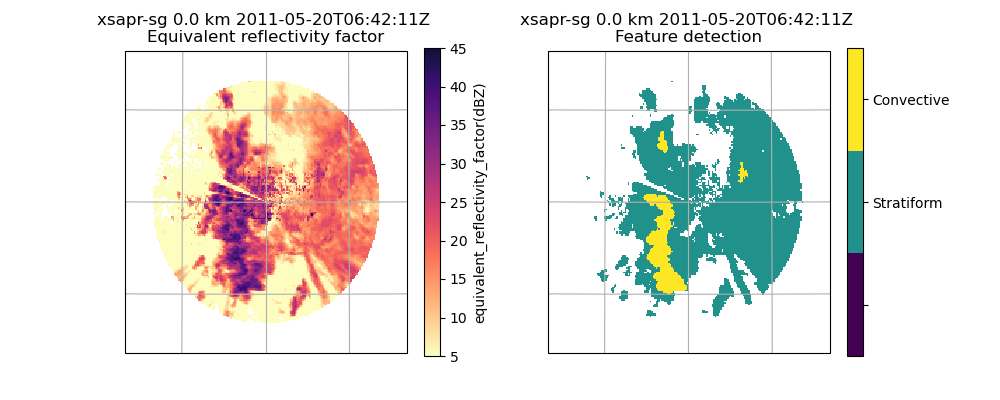## Summary of recommendations and best practices#

• Keep `estimate_flag=True` to see uncertainty in classification